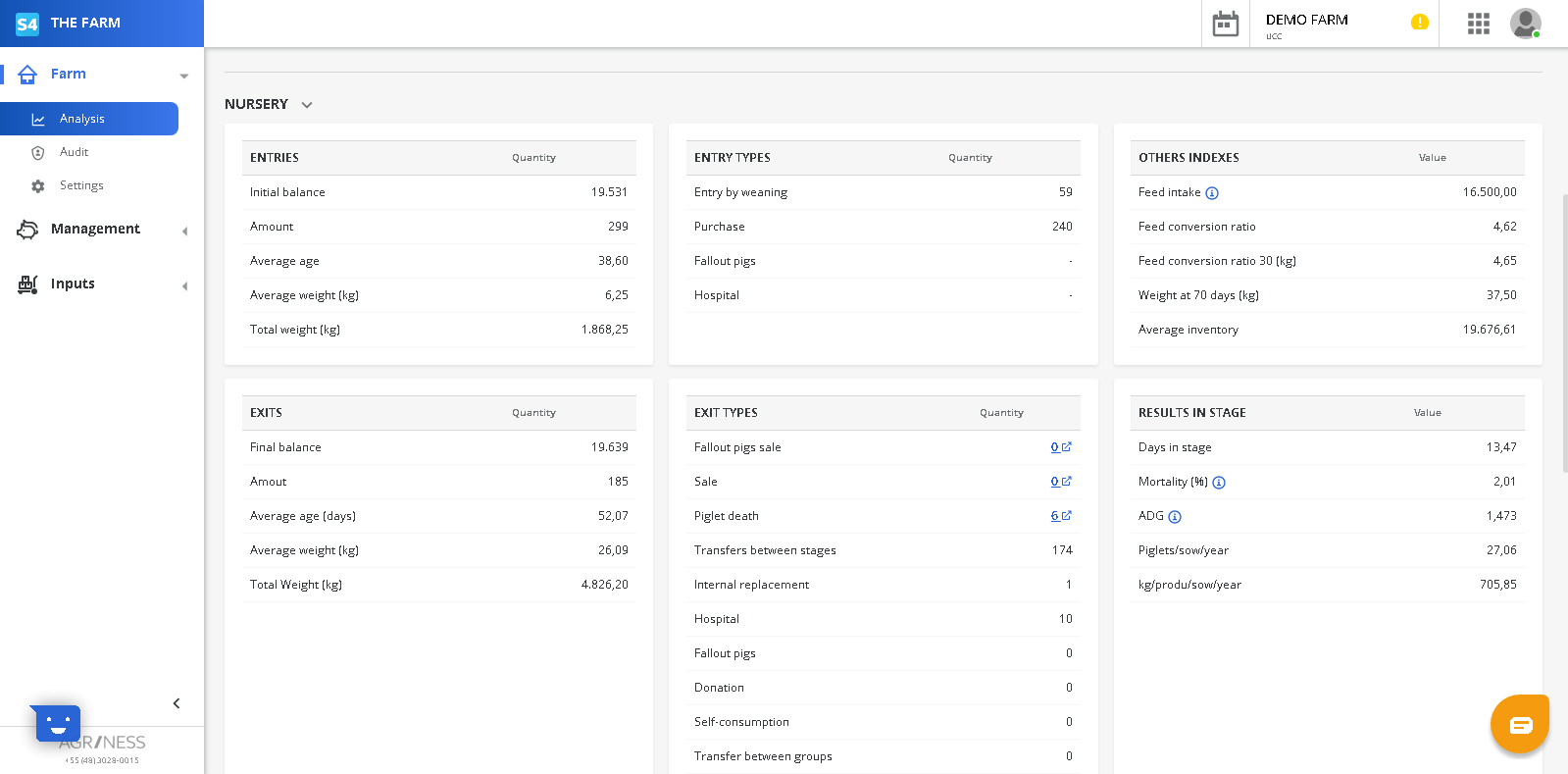Farms that has nursery and/or finishing has this specific part of the report  (farm performance) at: Farm > Analysis > Farm performance

Nursery:This report has six divisions:

• Entries
• Initial balance: quantity of animais one day before the Start date filtered
• Amount: total of piglets entered at the nursery
• Average age: Average age of piglets entered at this stage
• Average weight (kg):  Average weight of piglets entered at this stage
• Total weight (kg): Total weight of piglets entered at this stage
• Entry Types
• Entry by weaning: total of piglets entered by weaning
• Purchase: total of piglets purchased on the period
• Fallout pigs: total of piglets entered from another group
• Hospital: how many piglets entered from the hospital group
• Others Indexes
• Feed intake: total of feed consumed
• Feed conversation ratio: This represent how much of feed its necessary to produce 1 kg of pig
• Feed conversation ratio X (kg): It's how much food in kg you need to produce 1 kg of pig, where its adjustable in all groups by x weight of exit.
• Weight at Y days (kg): Average weight of the pigs on Y days, where (y) it's the estimated exit age of the pigs
• Average inventory: How many pigs the stage had on average
• Exits
• Final balance: Total of animals on the End Date filtered
• Amount:  How many pigs  left nursery or  left a group (can be by transfer between stages or groups, death, sale our another exit)
• Average weight (kg): Average exit weight of the pigs
• Total Weight (kg): Total exit weight of pigs
• Exit Types
• Fallout pig sales: how many pigs  left this stage by fallout pig sales
• Sale: quantity of pigs that left this stage by sale
• Piglet death: quantity of piglets death
• Transfer between stages: how many pigs were transferred  to finishing
• Internal replacement: how many  pigs were transferred to internal replacement
• Hospital: how many pigs left to the hospital group
• Fallout pigs: how many pigs left to the fallout group
• Donation: how many pigs were donated
• Self-consumption: how many pigs left from self-consumption
• Transfer between groups: how many pigs left from a transference between groups
• Results in Stage
• Days in stage: how many days ,on average, the pigs stay at this stage
• Mortality (%): How many  pigs died, in percentage
• ADG: It's on average how much the pigs weight gain per day (Average Daily Gain)
• Piglets/sow/year: how many pigs are produced per sow per year
• kg/produ/sow/year: how much weight of pig are produced per sow per year

Finishing:This report has six parts:

• Entries
• Initial balance: quantity of animais one day before  the Start date filtered
• Amount: total of pigs entered this stage
• Average age: Average age of pigs entered this stage
• Average weight (kg):  Average weight of pigs entered this stage
• Total weight (kg): Total weight of pigs entered this stage
• Entry Types
• Purchase: total of pigs purchased in period
• Transfer between stages: how many entered from nursery transference
• Fallout pigs: total of pigs entered from another group
• Hospital: how many pigs entered from the hospital group
• Transfer between groups: how many pigs entered from a transference between different groups
• Others Indexes
• Feed intake: total of feed consumed
• Feed conversation ratio: This represent how much of feed its necessary to produce 1 kg of pig
• Feed conversation ratio X (kg): It's how much of food in kg you need to produce 1 kg of pig, where its adjustable in all groups by x weight of exit.
• Weight at Y days (kg): Average weight of the pigs on Y days, where y it's the estimated exit age of the pigs
• Average inventory: How many pigs stay at this stage on average

• Exits
• Final balance: Total of animals at  the End Date filtered
• Amount: How many pigs left nursery or left a group (can be by transfer between stages or groups, death, sale our another exit)
• Average age (days): average age of pigs when they left the stage
• Average weight (kg): Average exit weight of the pigs
• Total Weight (kg): Total exit weight of pigs
• Exit Types
• Fallout pig sales: how many pigs left this stage by fallout  sales
• Sale: quantity of pigs that  left this stage by sale
• Piglet death: quantity of pigs death
• Fallout pigs: how many pigs left to the group from fallout
• Transfer between stages: how many pigs were transferred
• Internal replacement:  how many  pigs were transferred to internal replacement
• Hospital: how many pigs left to the hospital group
• Donation:  how many pigs were donated
• Self-consumption: how many pigs left to self-consumption
• Transfer between groups: groups: how many pigs left from to transference between groups
• Results in Stage
• Days in stage: how many days ,on average, the pigs stayed at this stage
• Mortality (%): How many  pigs died, in percentage
• ADG: It's on average how much the pigs weight gain per day (Average Daily Gain)
• Piglets/sow/year: how many pigs are produced per sow per year
• kg/produ/sow/year: how much weight of pig are produced per sow per year
• Sold/sow/Year: how many pigs sale (normal) per sow per year
• kg/Sold/Sow/Year: how many kilograms are sale (normal sale) per sow per year